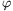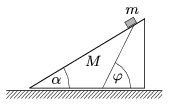Mathematical and Physical Journal
for High Schools
Issued by the MATFUND Foundation
 Already signed up? New to KöMaL?

#Problem P. 4277. (October 2010)

P. 4277. The wedge shown in the figure can slide without friction on a horizontal tabletop. The mass of the wedge is M and its angle of elevation is=30o. A body of mass m slides down without friction along the wedge, the path of the body makes an angle of=60o with the ground. Find the ratio of the masses, m/M.(5 pont)

Deadline expired on November 10, 2010.

Sorry, the solution is available only in Hungarian. Google translation

Megoldás.

$\displaystyle \frac{m}{M}=\frac{\tg60^\circ-\tg30^\circ}{\tg30^\circ}=2.$

### Statistics:

 113 students sent a solution. 5 points: 61 students. 4 points: 7 students. 3 points: 25 students. 2 points: 9 students. 1 point: 4 students. 0 point: 1 student. Unfair, not evaluated: 6 solutionss.

Problems in Physics of KöMaL, October 2010